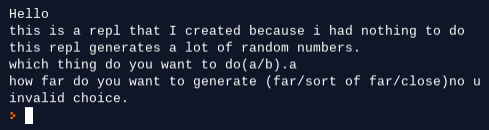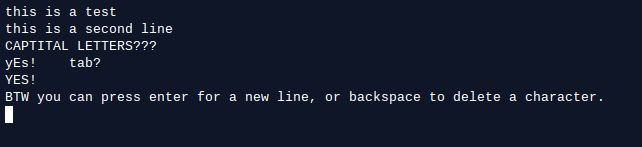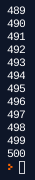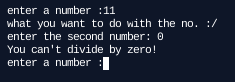# Allen Ding

@UniqueOstrich18 (290)
Check out my pinned repls!
posted to Share by UniqueOstrich18

@jeweledfox Sorry, I can't. Repl.it doesnt support the shift key for some reason.

how do you skip this?!?!?!??!??!

Replace line 16 with:

``  if generates == "far" or generates == "sort of far" or generates == "close": generate = input("press g to generate")``

And replace line 37 with:

``  if generates == "super far" or generates == "sort of super far" or generates == "far": generate = input("press g to generate")``Also, please check out this (https://repl.it/talk/share/2048-In-Python/31140). I spent a ton of time making it.

The package installation is failing because the package you are trying to install isn't supported by repl.it.

Space Race Game
posted to Share by blakethegreat

@MeharSinghSingh no you are

On line 60, change it to:

``  (''.join(choice((str.upper, str.lower))(c) for c in sentence3))``

Change line 56 to:

``"""``

Change line 61 to:

``"""``

Change line 41 to:

``  fortune1 = ''.join(choice((str.upper, str.lower))(c) for c in sentence1)``

It should work after you make those changes.

The fixed program is at https://repl.it/@UniqueOstrich18/Password-writer, if you just want to copy the code.

There are multiple fixes you need to make because I discovered more issues.

How to learn codes faster

Look in the learn section.

This happens when the system runs out of memory, and has to kill the program. Consider running it on your own computer, as repl.it only gives you 200MB of RAM.

Stopwatch Trouble plz help

Your issue is that you read the hour value from where the second value is stored, and vice versa. Change lines 7-14 to this:

``````with open("save.txt") as x:
for i, line in enumerate(x):
if i==0:
h=int(line)
if i==1:
m=int(line)
if i==2:
s=int(line)``````

How do you switch values

As seen on https://stackoverflow.com/questions/2493920/how-to-switch-position-of-two-items-in-a-python-list, you can just do this:

``thing[i], thing[j] = thing[j], thing[i]``

Where `i` is the index of your first item, and `j` is the index of your second item.

I need help with this code

@LOLLIPOP06 You can just put `print("The computer chose: " + computer)` before the if statements:

``````import random
computer = random.choice(["rock","paper","scissors"])value in the list
human_choice = input("choose rock, paper, or scissors: ")

print("The computer chose: " + computer) #<--- Add this code
if human_choice == 'rock'  and computer == 'rock':
print("This is draw!")
if human_choice == 'paper'  and computer == 'paper':
print("This is draw!")
if human_choice == 'scissors'  and computer == 'scissors':
print("This is draw!")
if human_choice == 'rock'  and computer == 'paper':
print("The computer won!")
if human_choice == 'rock'  and computer =='scissors':
print("You won!")
if human_choice == 'paper'  and computer =='rock':
print("You won!")
if human_choice == 'paper'  and computer == 'scissors':
print("The computer won!")
if human_choice == 'scissors'  and computer == 'rock':
print("The computer won!")
if human_choice == "scissors"  and computer == 'paper':
print("You won!")
if human_choice != "paper"  and human_choice != "scissors"  and human_choice != "rock":
print("You can only choose something from : ROCK PAPER OR SCISSORS")``````
I need help with this code

Your issue was that putting `computer = "rock" or "paper" or "scissors"` didn't actually do anything. You have to put `computer = random.choice(["rock","paper","scissors"])` Also, `input()` has to be assigned to a variable.

``````import random
computer = random.choice(["rock","paper","scissors"]) #random.choice takes in a list, then outputs a random value in the list
human_choice = input("choose rock, paper, or scissors: ") #you need to assign input() to a varible
#Your code below this works fine

if human_choice == 'rock'  and computer == 'rock':
print("This is draw!")
if human_choice == 'paper'  and computer == 'paper':
print("This is draw!")
if human_choice == 'scissors'  and computer == 'scissors':
print("This is draw!")
if human_choice == 'rock'  and computer == 'paper':
print("The computer won!")
if human_choice == 'rock'  and computer =='scissors':
print("You won!")
if human_choice == 'paper'  and computer =='rock':
print("You won!")
if human_choice == 'paper'  and computer == 'scissors':
print("The computer won!")
if human_choice == 'scissors'  and computer == 'rock':
print("The computer won!")
if human_choice == "scissors"  and computer == 'paper':
print("You won!")
if human_choice != "paper"  and human_choice != "scissors"  and human_choice != "rock":
print("You can only choose something from : ROCK PAPER OR SCISSORS")``````

WHY?

Yeah, that happened to me. They just fork my repls and remove the parts crediting me.

posted to Share by UniqueOstrich18

@Nathankang77 Also can you upvote this post?

Wack a Mole
posted to Share by AndrewZhao1

This is great

a clock
posted to Share by EricXie1

its a clock

``````       .-.-.
((  (__I__)  ))
.'_....._'.
/ / .12 . \ \
| | '  |  ' | |
| | 9  /  3 | |
\ \ '.6.' / /
'.`-...-'.'
/'-- --'\
`"""""""""```````

Clock ascii art from https://www.asciiart.eu/electronics/clocks

Import Variables from a file that isn't .py

The easiest way to store data in a file is using the `pickle` module. The `pickle` module stores, or "pickles," varibles and data in a file.

This is an example from the Python wiki:

``````# Save a dictionary into a pickle file.
import pickle

favorite_color = { "lion": "yellow", "kitty": "red" }

pickle.dump( favorite_color, open( "save.p", "wb" ) )``````
``````# Load the dictionary back from the pickle file.
import pickle

favorite_color = pickle.load( open( "save.p", "rb" ) )
# favorite_color is now { "lion": "yellow", "kitty": "red" }``````

@Axrevyn PyQt5 is a python module for the creation of guis.

It's just a repl.it thing. Same thing happened to PyQt5 a few months ago.

Does anyone have any project ideas?

Number guessing game?

Here's the fixed code:

``````import random
guesses = 0

while guesses<3:
value = random.randint(1, 10)
number_1 = input("\nEnter your guess (1-10): ")
if number_1.isdigit():
number_1 = int(number_1)
if number_1 == value:
print("You guessed the number!\nThe number generated was "+str(value))
break
else:
print("Wrong!\nThe number generated was "+str(value))
else:
print("Wrong!\nThe number generated was "+str(value))
guesses += 1``````

I've shortened the program to 17 lines:

``````print("Welcome to guess the number.")
print("There will be three rounds.")
print("This is round 1.")
print("(If your guess is incorrect run the code again.)")
print("I'm thinking of a number 1 through 10.")
def make_guess(number):
if number == guess:
print("Correct!")
else:
print("Incorrect...")
numbers = [7,4,6]
counter = 0
for number in numbers:
counter = counter + 1
print("Round "+ str(counter))
make_guess(number)``````

The output is exactly the same. The `make_guess` function takes the argument `number`, which will be used as the answer, and asks the user to guess. Then if the guess is equal to the answer, it prints "Correct!" If the guess is not equal to the answer, it prints "Incorrect..." The for loop at the end is so that the program can cycle through all of the answers in the list of answers. The counter variable is so that the program can keep track of what round it is.

Sorry if the text above is a little hard to understand.

Change line 23 and 24 to:

``main()``

There is no need for the if statement because the program is supposed to run the `main()` function anyways.

@Codemonkey51 Did you actually test that? If you just add `)` to the end of line 60, it won't work because there are more issues.

how do we print an apostrophe?

You can use `\` before the apostrophe, or use double quotes (`"`) to enclose the string that you want to print.

Backslash method:

``print('\'')``

Double quotes method:

``print("'")``

Typewriter Python

Here is a simple typewriter program, made in python 3:

``````import readchar,os
characters = []
def refresh():
os.system("clear")
string = ""
string = string.join(characters)
print(string)
while True:
try:
del characters[len(characters)-1]
except:
pass
characters.append("\n")
else:
characters.append(char)
refresh()``````

Output:You can run it at: https://repl.it/@UniqueOstrich18/Typewriter

Change:

``if elephant_weight > ant_weight``

To:

``if elephant_weight > ant_weight:``

If statements need to have `:` at the end of them.

Put an if statement where, if the counter is 501, then break out of the loop:

``````counter = 1

counter = int(input("what number do u want to count up from"))
while counter >=1:
print(counter)
counter = counter + 1
if counter == 501:
break``````Also, could you check out https://repl.it/talk/share/2048-In-Python/31140? I spent a lot of time making it.

how to fix zerodivisionerror

You can put the part where you divide the numbers in a `try: except:` block, so that when an error occurs, you can print a custom error. Also, you can half the program's length by using `or` in your if statements. The modified code is below.

``````from math import *

l = "\n"

while (1 == 1):
num1 = input("enter a number :")
vary = input("what you want to do with the no. :")
num2 = input("enter the second number: ")

while (1 == 1):
if vary == "+" or vary == "plus":
result = float(num1) + float(num2)
print(str(result) + str(l))
break
elif vary == "-" or vary == "minus":
result = float(num1) - float(num2)
print(str(result) + str(l))
break
elif vary == "*" or vary == "multiply" or vary == "x" or vary == "X":
result = float(num1) * float(num2)
print(str(result) + str(l))
break
elif vary == "/" or vary == "divide":
try:
result = float(num1)/float(num2) #if this part errors
print(str(result) + str(l))
except:
print("You can't divide by zero!") #then this part runs
break
elif vary == "power" or vary == "to the power" or vary == "^":
result = float(num1) ** float(num2)
print(str(result) + str(l))
break
else:
print("what you want to do with the no.s is not recoganized as an expression" + str(l))
break``````